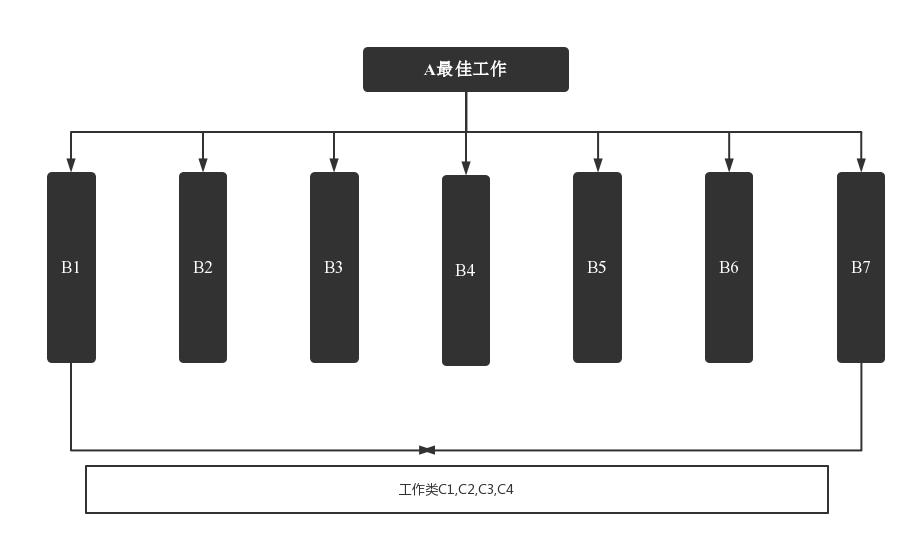• AHP层次分析法Python实现代码,如有需要，请大家下载！！！可用！！
• 数学建模——层次分析法Python代码 import numpy as np class AHP: “”" 相关信息的传入和准备 “”" def __init__(self, array): ## 记录矩阵相关信息 self.array = array ## 记录矩阵大小 self.n = array....
数学建模——层次分析法Python代码
import numpy as np class AHP: “”" 相关信息的传入和准备 “”"
def __init__(self, array):
## 记录矩阵相关信息
self.array = array
## 记录矩阵大小
self.n = array.shape
# 初始化RI值，用于一致性检验
self.RI_list = [0, 0, 0.52, 0.89, 1.12, 1.26, 1.36, 1.41, 1.46, 1.49, 1.52, 1.54, 1.56, 1.58,
1.59]
# 矩阵的特征值和特征向量
self.eig_val, self.eig_vector = np.linalg.eig(self.array)
# 矩阵的最大特征值
self.max_eig_val = np.max(self.eig_val)
# 矩阵最大特征值对应的特征向量
self.max_eig_vector = self.eig_vector[:, np.argmax(self.eig_val)].real
# 矩阵的一致性指标CI
self.CI_val = (self.max_eig_val - self.n) / (self.n - 1)
# 矩阵的一致性比例CR
self.CR_val = self.CI_val / (self.RI_list[self.n - 1])

"""
一致性判断
"""

def test_consist(self):
# 打印矩阵的一致性指标CI和一致性比例CR
print("判断矩阵的CI值为：" + str(self.CI_val))
print("判断矩阵的CR值为：" + str(self.CR_val))
# 进行一致性检验判断
if self.n == 2:  # 当只有两个子因素的情况
print("仅包含两个子因素，不存在一致性问题")
else:
if self.CR_val < 0.1:  # CR值小于0.1，可以通过一致性检验
print("判断矩阵的CR值为" + str(self.CR_val) + ",通过一致性检验")
return True
else:  # CR值大于0.1, 一致性检验不通过
print("判断矩阵的CR值为" + str(self.CR_val) + "未通过一致性检验")
return False

"""
算术平均法求权重
"""

def cal_weight_by_arithmetic_method(self):
# 求矩阵的每列的和
col_sum = np.sum(self.array, axis=0)
# 将判断矩阵按照列归一化
array_normed = self.array / col_sum
# 计算权重向量
array_weight = np.sum(array_normed, axis=1) / self.n
# 打印权重向量
print("算术平均法计算得到的权重向量为：\n", array_weight)
# 返回权重向量的值
return array_weight

"""
几何平均法求权重
"""

def cal_weight__by_geometric_method(self):
# 求矩阵的每列的积
col_product = np.product(self.array, axis=0)
# 将得到的积向量的每个分量进行开n次方
array_power = np.power(col_product, 1 / self.n)
# 将列向量归一化
array_weight = array_power / np.sum(array_power)
# 打印权重向量
print("几何平均法计算得到的权重向量为：\n", array_weight)
# 返回权重向量的值
return array_weight

"""
特征值法求权重
"""

def cal_weight__by_eigenvalue_method(self):
# 将矩阵最大特征值对应的特征向量进行归一化处理就得到了权重
array_weight = self.max_eig_vector / np.sum(self.max_eig_vector)
# 打印权重向量
print("特征值法计算得到的权重向量为：\n", array_weight)
# 返回权重向量的值
return array_weight

if name == “main”: # 给出判断矩阵 b = np.array([[1, 1 / 3, 1 / 8], [3, 1, 1 / 3], [8, 3, 1]])
# 算术平均法求权重
weight1 = AHP(b).cal_weight_by_arithmetic_method()
# 几何平均法求权重
weight2 = AHP(b).cal_weight__by_geometric_method()
# 特征值法求权重
weight3 = AHP(b).cal_weight__by_eigenvalue_method()展开全文数学建模
• 模糊层次分析法在方案优选方面的应用[J]. 计算机工程与设计,2004,25(10):1847-1849. DOI:10.3969/j.issn.1000-7024.2004.10.069. 根据假期数模训练题改编的python代码： from numpy import * B=[] print('指标...
由 陈欣. 模糊层次分析法在方案优选方面的应用[J]. 计算机工程与设计,2004,25(10):1847-1849. DOI:10.3969/j.issn.1000-7024.2004.10.069.
根据假期数模训练题改编的python代码，自己改美观点吧：from numpy import *
B=[]
print('指标排名:')
for i in range(0,8):
B.append(int(input()))
S=zeros(shape=(8,8))
for i in range(0,len(B)):
for j in range(i,len(B)):
if B[i]==B[j]:
S[i][j]=0.5
S[j][i]=0.5
if B[i]>B[j]:
S[i][j]=0
S[j][i]=1
if B[i]<B[j]:
S[i][j]=1
S[j][i]=0
print("A-B优先关系矩阵：")
print(S)
#B-C优先关系矩阵
C=*8
C=array([[0.5,0,0,1],[1,0.5,0,1],[1,1,0.5,1],[0,0,0,0.5]])
C=array([[0.5,0,0,0],[1,0.5,0,0],[1,1,0.5,0],[1,1,1,0.5]])
C=array([[0.5,1,1,1],[0,0.5,0,1],[0,1,0.5,1],[0,0,0,0.5]])
C=array([[0.5,1,0,0],[0,0.5,0,0],[1,1,0.5,1],[1,1,0,0.5]])
C=array([[0.5,1,1,1],[0,0.5,1,1],[0,0,0.5,0],[0,0,1,0.5]])
C=array([[0.5,1,1,1],[0,0.5,0,1],[0,1,0.5,1],[0,0,0,0.5]])
C=array([[0.5,0,1,1],[1,0.5,1,1],[0,0,0.5,1],[0,0,0,0.5]])
C=array([[0.5,0,0,0],[1,0.5,0,0],[1,1,0.5,0],[1,1,1,0.5]])
#转换成模糊一致矩阵
S=array(S)
p=S.sum(axis=1)
for i in range(0,8):
for j in range(0,8):
S[i][j]=(p[i]-p[j])/(8*2)+0.5
print('A-B模糊一致矩阵：')
print(S)
print('B-C模糊一致矩阵:')
for i in range(0,8):
r=C[i].sum(axis=1)
for j in range(0,4):
for k in range(0,4):
C[i][j][k]=(r[j]-r[k])/(4*2)+0.5
print(C[i])
#层次单排序
su=*8
for i in range(0,8):
for j in range(0,8):
su[i]*=S[i][j]
su[i]=su[i]**(1/8)
SU=sum(su)
su=array(su)
w0=su/SU
print('各指标权重:')
print(w0)
w=[]
for i in range(0,8):
zu=*4
for j in range(0,4):
for k in range(0,4):
zu[j]*=C[i][j][k]
zu[j]=zu[j]**(1/4)
ZU=sum(zu)
zu=array(zu)
w.append(zu/ZU)
print(w[i])
#层次总排序
w0=array(w0)
w=array(w)
y=dot(w0.T,w)
print('总分：')
y=y.T
m=dict()
n=['C1','C2','C3','C4']
for i in range(0,4):
m[n[i]]=y[i]
print(m)


展开全文• python实现三种方法求权重
用python实现三种方法求权重
import numpy as np

A = np.array() #输入判断矩阵
(n,n) = np.shape(A)

# 算术平均法求权重
sum_1 = A.sum(axis=0) #对每一列求和
B = A/sum_1  #归一化处理
sum_2 = B.sum(axis=1) #对每一行求和
suan = sum_2/n #除N得权重
print('算术平均法所得权重为：')
print(suan)
print('\n')

#几何平均法求权重
multiply_1 = A.prod(axis=1) #按行相乘
C = np.power(multiply_1,1/n) # 开n次方
sum_1 = C.sum(axis=0)
jihe = C /sum_1
print('几何平均法所得权重为：')
print(jihe)
print('\n')

#特征值法求权重
D = np.linalg.eig(A)
max_eig = np.max(D) #最大特征值
position = np.argmax(D) #最大特征值位置
E = D  #特征向量
E = E[:,position]
tezheng = E/sum(E) #归一化处理
print('特征法所求权重为：')
print(tezheng)
print('\n')

#计算一致性比例
CI = (max_eig - n) / (n-1)
RI = [0,0,0.52,0.89,1.12,1.26,1.36,1.41,1.46,1.49,1.52,1.54,1.56,1.58,1.59]
CR = CI / RI[n-1]
print('一致性指标CI：')
print(CI)
print('一致性比例CR：')
print(CR)
if CR<0.1:
print('一致性可以接受')
else:
print('一致性不可接受')

求得权重用excel进行计算会方便很多
展开全文数学建模 numpy
• 某高校正在进行教师的评优工作，现应用层次分析法对待评教师的综合素质进行评价。整个层次结构分为三层，最高层即问题分析的总目标，要评选出优秀教师A；第二层是准则层，包括三种指标学识水平C1、科研能力C2、教学...
问题背景描述 某高校正在进行教师的评优工作，现应用层次分析法对待评教师的综合素质进行评价。整个层次结构分为三层，最高层即问题分析的总目标，要评选出优秀教师A；第二层是准则层，包括三种指标学识水平C1、科研能力C2、教学工作C3；第三层是方案层，即参加评优的教师，假设对五位候选教师P1、P2、P3、P4、P5进行评优，其中P2、P3、P4为任课教师，需要从学识水平、科研能力、教学工作三方面评估其综合素质，教师P5是科研人员，只需从学识水平、科研能力两方面衡量其综合素质，教师P1是行政人员，只需从学识水平和教学工作两方面衡量。 三个评价指标的相对重要程度不同，并且各位教师在三个指标上表现不同，具体如下：科研能力指标比学识水平指标明显重要，教学工作指标比学识水平指标稍微重要，科研能力指标比教学工作指标稍微重要。在学识水平上，P1稍微高于P2，P1明显高于P3，P1比P4处于稍微高与明显高之间，P1强烈高于P5，P2稍微高于P3，P2比P4处于同样高与稍微高之间，P2明显高于P5，P3比P5处于同样高与稍微高之间，P4比P3处于同样高与稍微高之间，P4稍微高于P5；在科研能力上，P3强烈高于P2，P4稍微高于P2，P5明显高于P2，P3明显高于P4，P3比P5处于同样高与稍微高之间，P5稍微高于P4；在教学工作上，P1稍微高于P3，P1稍微高于P4，P2稍微高于P3，P2稍微高于P4，P1与P2水平相当，P3与P4水平相当。
import numpy as np  # Python本身没有处理矩阵的数据类型，因此需要使用附加的函数库。

"""
1. 读取csv文件
"""

def prin(path):
tmp = tmp.astype(float)  # 类型转换
return tmp

"""
2. 方根法求单层元素相对重要性
"""

def ReImpo(F):
n = np.shape(F)  # F矩阵的行数，np.shape(F)为列数
W = np.zeros([1, n])  # 生成1行n列的0矩阵
for i in range(n):
t = 1
for j in range(n):
t = F[i, j] * t  # 累乘
W[0, i] = t ** (1 / n)  # 开n次方根求Wi
W = W / sum(W[0, :])  # 归一化
return W.T

"""
3. 一致性检验
"""

def isConsist(F, RI):
n = np.shape(F)  # F矩阵的行数，np.shape(F)为列数
W = np.zeros([1, n])
λmi = np.zeros([1, n])
λmax = 0
for i in range(n):
t = 1
for j in range(n):
t = F[i, j] * t  # 累乘
W[0, i] = t ** (1 / n)  # 开n次方根求Wi
for i in range(n):
temp = 0
for j in range(n):
temp = F[i, j] * W[0, j] + temp
λmi[0, i] = temp / W[0, i]
λmax = λmax + λmi[0, i] / n
CI = (λmax - n) / (n - 1)
CR = CI / RI
if CR <= 0.1:
return bool(1)
else:
return bool(0)

"""
4. 计算综合重要性
"""

def ComImpo(F12, F231, F232, F233):  # 综合重要性
if isConsist(F12, 0.58) and isConsist(F231, 1.12) and isConsist(F232, 0.90) and isConsist(F233, 0.90):
W12 = ReImpo(F12)
W231 = ReImpo(F231)
b = np.array([])
W232 = np.r_[b, ReImpo(F232)]  # np.r_是按列连接两个矩阵
W233 = np.r_[ReImpo(F233), b]
W23 = np.hstack([W231, W232, W233])  # np.hstack将参数元组的元素数组按水平方向进行叠加
else:
print("判断矩阵不通过一致性检验！")
return 0
n = len(W23)
C = np.zeros([1, n])
for i in range(n):
t = W23[i, :]
C[0, i] = sum((W12.T * t))
return C

def main():
F1 = prin("F1.csv")
F2 = prin("F2.csv")
F3 = prin("F3.csv")
F4 = prin("F4.csv")
C = ComImpo(F1, F2, F3, F4)
print("P1-P5五位教师综合推荐指数分别为：")
for i in range(5):
print(C[0, i])
print("因此评价排序为：")
print(np.argsort(-C) + 1)  # 将C中的元素从大到小排列，提取其对应的index(索引)

if __name__ == '__main__':
main()


相对于总目标各指标间的相对重要性（三阶判断矩阵）各教师的学识水平比较（五阶判断矩阵）各教师的科研能力比较（四阶判断矩阵）各教师的教学工作比较（四阶判断矩阵）运行结果实验结果表明，对P1-P5五位教师综合素质进行评价排序由高到低为：P3,P5,P2,P1,P4。
展开全文人工智能
• 使用Python语言实现AHP算法，运行代码需预先安装numpy包，Python3以上版本
• 这里用python语言来计算判断矩阵的权重，网上大部分是matlab语言，里面也包含一致性检验的函数，具体各函数使用方法详见代码注释的部分 import numpy as np a=np.array([[1, 1 / 4, 2, 1 / 3], [4, 1, 8, 2], [1 / 2...数学建模
• 目录层次分析法概述定义步骤归纳例子应用实例Python实现程序如下：运行结果截图 层次分析法概述 定义 本文所有图片均来自本人的OneNote笔记 步骤归纳 例子 建立层次结构模型 构造判断(成对比较)矩阵 第...
• 层次分析法代码，可以通过该代码进行适当改变，针对自己的问题进行层次分析，采用python
• 层次分析法根据问题的性质和要达到的总目标，将问题分解为不同的组成因素，并按照因素间的相互关联影响以及隶属关系将因素按不同层次聚集组合，形成–个多层次的分析结构模型,从而最终使问题归结为最低层(供决策的...
• Python实现“层次分析法”及“自调节层次分析法” 假设我们遇到如下问题： ①对于M个方案，每个方案有N个属性，在已知各个方案每个属性值&&任意两个属性的重要程度的前提下，如何选择最优的方案？ ②对于一...算法
• AHP (Analytic Hierarchy Process)层次分析法是一种实用的多方案或多目标的决策方法。 层次分析法是一种主客观结合的多指标综合评价方法；即定性分析与定量分析有机结合，实现定量化决策。 有两次定权过程，即...
• 层次分析法（The analytic hierarchy process）简称AHP 在20世纪70年代中期由美国运筹学家托马斯·塞蒂（T.L.saaty）正式提出。它是一种定性和定量相结合的、系统化、层次化的分析方法。由于它在处理复杂的决策问题...数学建模
• import numpy as np #根据问题更改 importance = np.array([[1, 1 / 3, 1, 1 / 5, 2, 3], #这里输入构造矩阵 [3, 1, 2, 1 / 5, 3, 4], [1, 1 / 2, 1, 1 / 4, 3, 2], [5, 5, 4, 1, 5, 6], [1 / 2, 1 / 3, 1 / 3,...python
• 最近在做一个集群的项目，涉及到负载均衡问题，其中节点的综合负载评价部分采用经典的层次分析法，项目需要用C++复现。鉴于Python成熟、方便的矩阵操作，先用Python简单复现一下该算法。 1 理论基础 关于AHP层次分析...
• 一、层次分析法原理 层次分析法（Analytic Hierarchy Process，AHP）由美国运筹学家托马斯·塞蒂（T. L. Saaty）于20世纪70年代中期提出，用于确定评价模型中各评价因子/准则的权重，进一步选择最优方案。该方法仍...
• 随机一致性指标为0.0993908598585786，判断矩阵具有满意的一致性。 最大的特征值为： 6.616223331123187 对应的特征向量为： [0.083 0.475 0.205 0.108 0.065 0.065] 各指标权重为：...python
• 模糊综合评价（FCE）是一种根据模糊数学隶属度理论把定性评价转化为定量评价的方法，它具有结果清晰，系统性强的特点，能较好地解决模糊的、难以量化的问题，适合各种非确定性问题的解决。我们先看模糊综合评价...大数据 考核评价
• python实现层次分析法(AHP） 层次分析法是数学建模的入门算法，类似于算法竞赛中的cout<<"Hello,world!"<<endl; 对于python数据分析的初学者来说，在使用python实现层次分析法时，难点是特征值法的...numpy 数学建模
• 层次分析法（The analytic hierarchy process）简称AHP，它是将与决策有关的因素分解成目标、准则、方案等层次，在此基础之上进行定性和定量分析的决策方法。 本文为简明AHP学习笔记，并通过Python实践构建多层权重...决策分析
• 一、层次分析法原理 层次分析法（Analytic Hierarchy Process，AHP）由美国运筹学家托马斯·塞蒂（T. L. Saaty）于20世纪70年代中期提出，用于确定评价模型中各评价因子/准则的权重，进一步选择最优方案。该方法仍......

# 层次分析法pythonpython 订阅